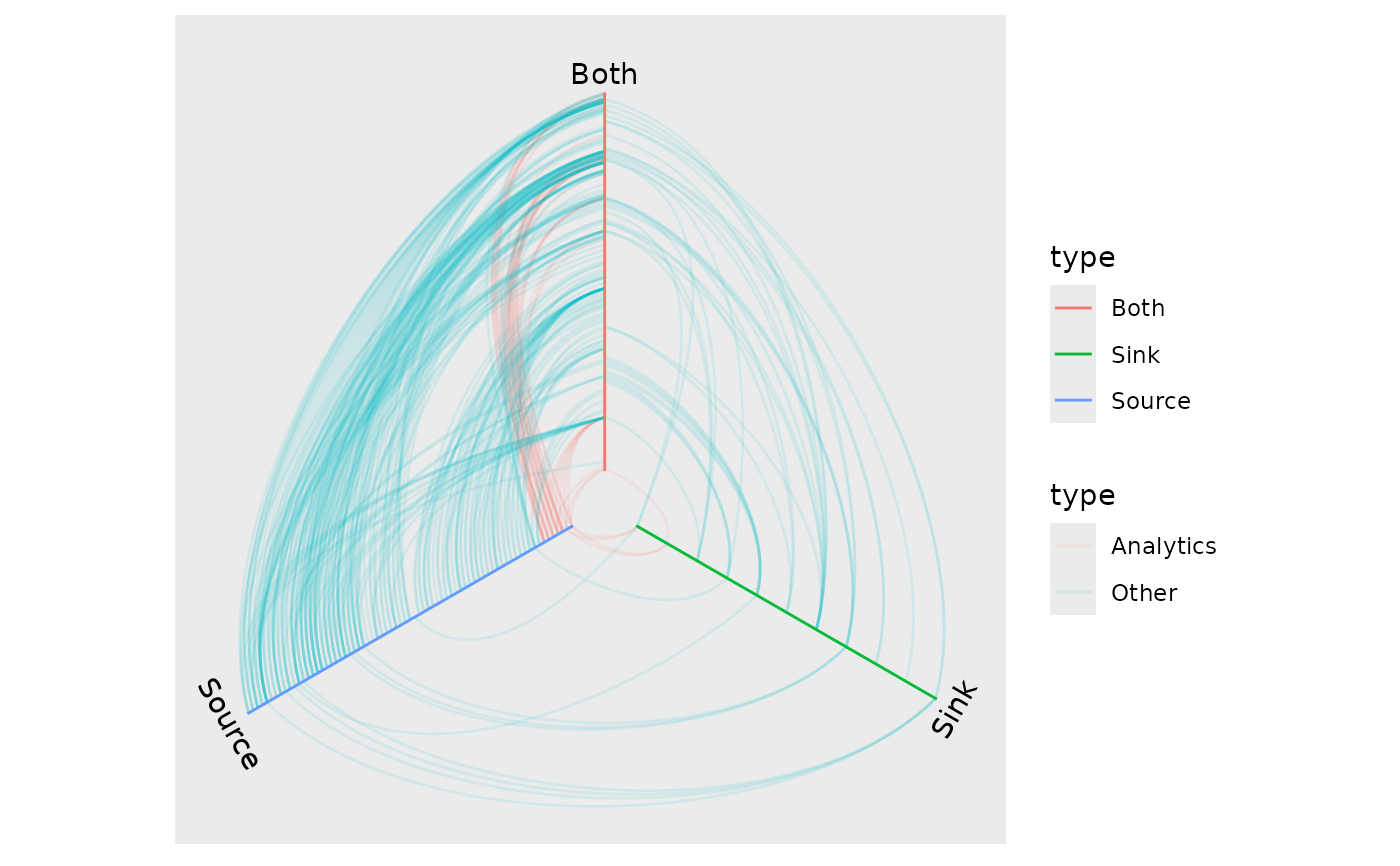This function lets you annotate the axes in a hive plot with labels and color coded bars.

geom_axis_hive(
mapping = NULL,
data = NULL,
position = "identity",
label = TRUE,
axis = TRUE,
show.legend = NA,
...
)

## Arguments

mapping

Set of aesthetic mappings created by aes() or aes_(). If specified and inherit.aes = TRUE (the default), it is combined with the default mapping at the top level of the plot. You must supply mapping if there is no plot mapping.

data

The data to be displayed in this layer. There are three options:

If NULL, the default, the data is inherited from the plot data as specified in the call to ggplot().

A data.frame, or other object, will override the plot data. All objects will be fortified to produce a data frame. See fortify() for which variables will be created.

A function will be called with a single argument, the plot data. The return value must be a data.frame, and will be used as the layer data. A function can be created from a formula (e.g. ~ head(.x, 10)).

position

Position adjustment, either as a string, or the result of a call to a position adjustment function.

label

Should the axes be labelled. Defaults to TRUE

axis

Should a rectangle be drawn along the axis. Defaults to TRUE

show.legend

logical. Should this layer be included in the legends? NA, the default, includes if any aesthetics are mapped. FALSE never includes, and TRUE always includes. It can also be a named logical vector to finely select the aesthetics to display.

...

Other arguments passed on to layer(). These are often aesthetics, used to set an aesthetic to a fixed value, like colour = "red" or size = 3. They may also be parameters to the paired geom/stat.

## Aesthetics

geom_axis_hive understand the following aesthetics.

• alpha

• colour

• fill

• size

• linetype

• label_size

• family

• fontface

• lineheight

## Author

Thomas Lin Pedersen

## Examples

# Plot the flare import graph as a hive plot
library(tidygraph)
flareGr <- as_tbl_graph(flare$imports) %>% mutate( type = dplyr::case_when( centrality_degree(mode = 'in') == 0 ~ 'Source', centrality_degree(mode = 'out') == 0 ~ 'Sink', TRUE ~ 'Both' ) ) %>% activate(edges) %>% mutate( type = dplyr::case_when( grepl('flare.analytics', paste(.N()$name[from], .N()\$name[to])) ~ 'Analytics',
TRUE ~ 'Other'
)
)
ggraph(flareGr, 'hive', axis = type) +
geom_edge_hive(aes(colour = type), edge_alpha = 0.1) +
geom_axis_hive(aes(colour = type)) +
coord_fixed()